Given the importance of learning rate and the learning rate schedule for training neural networks, there have been a number of research papers published recently on the subject. Although many practitioners are using simple learning rate schedules such as stepwise decay, research has shown that there are other strategies that work better in most situations. We implement a number of different schedule shapes in this tutorial and introduce cyclical schedules.

See the “Learning Rate Schedules” tutorial for a more basic overview of learning rates, and an example of how to use them while training your own models.

%matplotlib inline
import copy
import math
import mxnet as mx
import numpy as np
import matplotlib.pyplot as plt

def plot_schedule(schedule_fn, iterations=1500):
# Iteration count starting at 1
iterations = [i+1 for i in range(iterations)]
lrs = [schedule_fn(i) for i in iterations]
plt.scatter(iterations, lrs)
plt.xlabel("Iteration")
plt.ylabel("Learning Rate")
plt.show()


## Custom Schedule Shapes¶

### (Slanted) Triangular¶

While trying to push the boundaries of batch size for faster training, Priya Goyal et al. (2017) found that having a smooth linear warm up in the learning rate at the start of training improved the stability of the optimizer and lead to better solutions. It was found that a smooth increases gave improved performance over stepwise increases.

We look at “warm-up” in more detail later in the tutorial, but this could be viewed as a specific case of the “triangular” schedule that was proposed by Leslie N. Smith (2015). Quite simply, the schedule linearly increases then decreases between a lower and upper bound. Originally it was suggested this schedule be used as part of a cyclical schedule but more recently researchers have been using a single cycle.

One adjustment proposed by Jeremy Howard, Sebastian Ruder (2018) was to change the ratio between the increasing and decreasing stages, instead of the 50:50 split. Changing the increasing fraction (inc_fraction!=0.5) leads to a “slanted triangular” schedule. Using inc_fraction<0.5 tends to give better results.

class TriangularSchedule():
def __init__(self, min_lr, max_lr, cycle_length, inc_fraction=0.5):
"""
min_lr: lower bound for learning rate (float)
max_lr: upper bound for learning rate (float)
cycle_length: iterations between start and finish (int)
inc_fraction: fraction of iterations spent in increasing stage (float)
"""
self.min_lr = min_lr
self.max_lr = max_lr
self.cycle_length = cycle_length
self.inc_fraction = inc_fraction

def __call__(self, iteration):
if iteration <= self.cycle_length*self.inc_fraction:
unit_cycle = iteration * 1 / (self.cycle_length * self.inc_fraction)
elif iteration <= self.cycle_length:
unit_cycle = (self.cycle_length - iteration) * 1 / (self.cycle_length * (1 - self.inc_fraction))
else:
unit_cycle = 0
adjusted_cycle = (unit_cycle * (self.max_lr - self.min_lr)) + self.min_lr


We look an example of a slanted triangular schedule that increases from a learning rate of 1 to 2, and back to 1 over 1000 iterations. Since we set inc_fraction=0.2, 200 iterations are used for the increasing stage, and 800 for the decreasing stage. After this, the schedule stays at the lower bound indefinitely.

schedule = TriangularSchedule(min_lr=1, max_lr=2, cycle_length=1000, inc_fraction=0.2)
plot_schedule(schedule)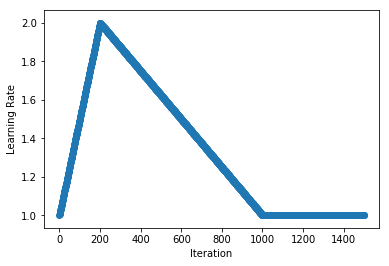### Cosine¶

Continuing with the idea that smooth decay profiles give improved performance over stepwise decay, Ilya Loshchilov, Frank Hutter (2016) used “cosine annealing” schedules to good effect. As with triangular schedules, the original idea was that this should be used as part of a cyclical schedule, but we begin by implementing the cosine annealing component before the full Stochastic Gradient Descent with Warm Restarts (SGDR) method later in the tutorial.

class CosineAnnealingSchedule():
def __init__(self, min_lr, max_lr, cycle_length):
"""
min_lr: lower bound for learning rate (float)
max_lr: upper bound for learning rate (float)
cycle_length: iterations between start and finish (int)
"""
self.min_lr = min_lr
self.max_lr = max_lr
self.cycle_length = cycle_length

def __call__(self, iteration):
if iteration <= self.cycle_length:
unit_cycle = (1 + math.cos(iteration * math.pi / self.cycle_length)) / 2
adjusted_cycle = (unit_cycle * (self.max_lr - self.min_lr)) + self.min_lr
else:
return self.min_lr


We look at an example of a cosine annealing schedule that smoothing decreases from a learning rate of 2 to 1 across 1000 iterations. After this, the schedule stays at the lower bound indefinietly.

schedule = CosineAnnealingSchedule(min_lr=1, max_lr=2, cycle_length=1000)
plot_schedule(schedule)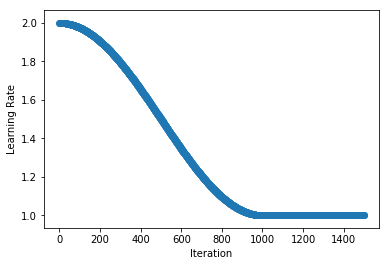## Custom Schedule Modifiers¶

We now take a look some adjustments that can be made to existing schedules. We see how to add linear warm-up and its compliment linear cool-down, before using this to implement the “1-Cycle” schedule used by Leslie N. Smith, Nicholay Topin (2017) for “super-convergence”. We then look at cyclical schedules and implement the original cyclical schedule from Leslie N. Smith (2015) before finishing with a look at “SGDR: Stochastic Gradient Descent with Warm Restarts” by Ilya Loshchilov, Frank Hutter (2016).

Unlike the schedules above and those implemented in mx.lr_scheduler, these classes are designed to modify existing schedules so they take the argument schedule (for initialized schedules) or schedule_class when being initialized.

### Warm-Up¶

Using the idea of linear warm-up of the learning rate proposed in “Accurate, Large Minibatch SGD: Training ImageNet in 1 Hour” by Priya Goyal et al. (2017), we implement a wrapper class that adds warm-up to an existing schedule. Going from start_lr to the initial learning rate of the schedule over length iterations, this adjustment is useful when training with large batch sizes.

class LinearWarmUp():
def __init__(self, schedule, start_lr, length):
"""
schedule: a pre-initialized schedule (e.g. TriangularSchedule(min_lr=0.5, max_lr=2, cycle_length=500))
start_lr: learning rate used at start of the warm-up (float)
length: number of iterations used for the warm-up (int)
"""
self.schedule = schedule
self.start_lr = start_lr
# calling mx.lr_scheduler.LRScheduler effects state, so calling a copy
self.finish_lr = copy.copy(schedule)(0)
self.length = length

def __call__(self, iteration):
if iteration <= self.length:
return iteration * (self.finish_lr - self.start_lr)/(self.length) + self.start_lr
else:
return self.schedule(iteration - self.length)


As an example, we add a linear warm-up of the learning rate (from 0 to 1 over 250 iterations) to a stepwise decay schedule. We first create the MultiFactorScheduler (and set the base_lr) and then pass it to LinearWarmUp to add the warm-up at the start. We can use LinearWarmUp with any other schedule including CosineAnnealingSchedule.

schedule = mx.lr_scheduler.MultiFactorScheduler(step=[250, 750, 900], factor=0.5)
schedule.base_lr = 1
schedule = LinearWarmUp(schedule, start_lr=0, length=250)
plot_schedule(schedule)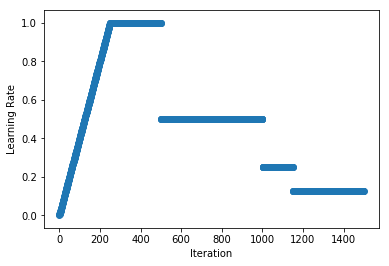### Cool-Down¶

Similarly, we could add a linear cool-down period to our schedule and this is used in the “1-Cycle” schedule proposed by Leslie N. Smith, Nicholay Topin (2017) to train neural networks very quickly in certain circumstances (coined “super-convergence”). We reduce the learning rate from its value at start_idx of schedule to finish_lr over a period of length, and then maintain finish_lr thereafter.

class LinearCoolDown():
def __init__(self, schedule, finish_lr, start_idx, length):
"""
schedule: a pre-initialized schedule (e.g. TriangularSchedule(min_lr=0.5, max_lr=2, cycle_length=500))
finish_lr: learning rate used at end of the cool-down (float)
start_idx: iteration to start the cool-down (int)
length: number of iterations used for the cool-down (int)
"""
self.schedule = schedule
# calling mx.lr_scheduler.LRScheduler effects state, so calling a copy
self.start_lr = copy.copy(self.schedule)(start_idx)
self.finish_lr = finish_lr
self.start_idx = start_idx
self.finish_idx = start_idx + length
self.length = length

def __call__(self, iteration):
if iteration <= self.start_idx:
return self.schedule(iteration)
elif iteration <= self.finish_idx:
return (iteration - self.start_idx) * (self.finish_lr - self.start_lr) / (self.length) + self.start_lr
else:
return self.finish_lr


As an example, we apply learning rate cool-down to a MultiFactorScheduler. Starting the cool-down at iteration 1000, we reduce the learning rate linearly from 0.125 to 0.001 over 500 iterations, and hold the learning rate at 0.001 after this.

schedule = mx.lr_scheduler.MultiFactorScheduler(step=[250, 750, 900], factor=0.5)
schedule.base_lr = 1
schedule = LinearCoolDown(schedule, finish_lr=0.001, start_idx=1000, length=500)
plot_schedule(schedule)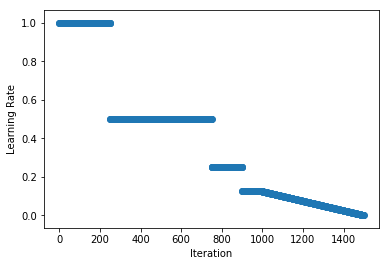#### 1-Cycle: for “Super-Convergence”¶

So we can implement the “1-Cycle” schedule proposed by Leslie N. Smith, Nicholay Topin (2017) we use a single and symmetric cycle of the triangular schedule above (i.e. inc_fraction=0.5), followed by a cool-down period of cooldown_length iterations.

class OneCycleSchedule():
def __init__(self, start_lr, max_lr, cycle_length, cooldown_length=0, finish_lr=None):
"""
start_lr: lower bound for learning rate in triangular cycle (float)
max_lr: upper bound for learning rate in triangular cycle (float)
cycle_length: iterations between start and finish of triangular cycle: 2x 'stepsize' (int)
cooldown_length: number of iterations used for the cool-down (int)
finish_lr: learning rate used at end of the cool-down (float)
"""
if (cooldown_length > 0) and (finish_lr is None):
raise ValueError("Must specify finish_lr when using cooldown_length > 0.")
if (cooldown_length == 0) and (finish_lr is not None):
raise ValueError("Must specify cooldown_length > 0 when using finish_lr.")

finish_lr = finish_lr if (cooldown_length > 0) else start_lr
schedule = TriangularSchedule(min_lr=start_lr, max_lr=max_lr, cycle_length=cycle_length)
self.schedule = LinearCoolDown(schedule, finish_lr=finish_lr, start_idx=cycle_length, length=cooldown_length)

def __call__(self, iteration):
return self.schedule(iteration)


As an example, we linearly increase and then decrease the learning rate from 0.1 to 0.5 and back over 500 iterations (i.e. single triangular cycle), before reducing the learning rate further to 0.001 over the next 750 iterations (i.e. cool-down).

schedule = OneCycleSchedule(start_lr=0.1, max_lr=0.5, cycle_length=500, cooldown_length=750, finish_lr=0.001)
plot_schedule(schedule)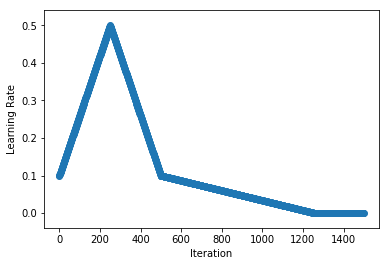### Cyclical¶

Originally proposed by Leslie N. Smith (2015), the idea of cyclically increasing and decreasing the learning rate has been shown to give faster convergence and more optimal solutions. We implement a wrapper class that loops existing cycle-based schedules such as TriangularSchedule and CosineAnnealingSchedule to provide infinitely repeating schedules. We pass the schedule class (rather than an instance) because one feature of the CyclicalSchedule is to vary the cycle_length over time as seen in Ilya Loshchilov, Frank Hutter (2016) using cycle_length_decay. Another feature is the ability to decay the cycle magnitude over time with cycle_magnitude_decay.

class CyclicalSchedule():
def __init__(self, schedule_class, cycle_length, cycle_length_decay=1, cycle_magnitude_decay=1, **kwargs):
"""
schedule_class: class of schedule, expected to take cycle_length argument
cycle_length: iterations used for initial cycle (int)
cycle_length_decay: factor multiplied to cycle_length each cycle (float)
cycle_magnitude_decay: factor multiplied learning rate magnitudes each cycle (float)
kwargs: passed to the schedule_class
"""
self.schedule_class = schedule_class
self.length = cycle_length
self.length_decay = cycle_length_decay
self.magnitude_decay = cycle_magnitude_decay
self.kwargs = kwargs

def __call__(self, iteration):
cycle_idx = 0
cycle_length = self.length
idx = self.length
while idx <= iteration:
cycle_length = math.ceil(cycle_length * self.length_decay)
cycle_idx += 1
idx += cycle_length
cycle_offset = iteration - idx + cycle_length

schedule = self.schedule_class(cycle_length=cycle_length, **self.kwargs)
return schedule(cycle_offset) * self.magnitude_decay**cycle_idx


As an example, we implement the triangular cyclical schedule presented in “Cyclical Learning Rates for Training Neural Networks” by Leslie N. Smith (2015). We use slightly different terminology to the paper here because we use cycle_length that is twice the ‘stepsize’ used in the paper. We repeat cycles, each with a length of 500 iterations and lower and upper learning rate bounds of 0.5 and 2 respectively.

schedule = CyclicalSchedule(TriangularSchedule, min_lr=0.5, max_lr=2, cycle_length=500)
plot_schedule(schedule)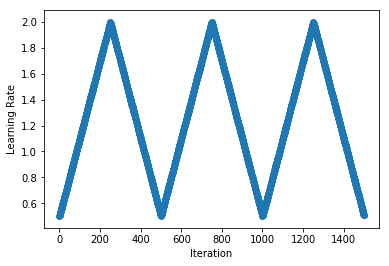And lastly, we implement the scheduled used in “SGDR: Stochastic Gradient Descent with Warm Restarts” by Ilya Loshchilov, Frank Hutter (2016). We repeat cosine annealing schedules, but each time we halve the magnitude and double the cycle length.

schedule = CyclicalSchedule(CosineAnnealingSchedule, min_lr=0.01, max_lr=2,
cycle_length=250, cycle_length_decay=2, cycle_magnitude_decay=0.5)
plot_schedule(schedule)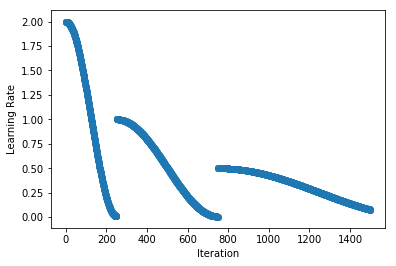Want to learn more? Checkout the “Learning Rate Schedules” tutorial for a more basic overview of learning rates found in mx.lr_scheduler, and an example of how to use them while training your own models.